• 50
•
• 6
•
•
•
•
•
56
Shares

# RESISTANCE AND RESISTIVITY

### INTRODUCTION

See, resistance is everywhere. Everything which is in our surroundings stop us do new things. So this acts like a resistance. Resistance means, anything which resist ours action.

This is not only seen in electrical wires rather it also seen in our daily life. For example, When you drive bycycle in the opposite direction of blowing wind then you must have experience that you have to apply more effort to keep your balance on your bycycle.

This wind lower your speed because you are going in opposite of that blowing wind. So from this, we can conclude that if anyone goes opposite of any rules whether it is the rule of physics or something else, then they have to face restrictions, this restrictions is nothing but only the resistance.

In this article, we are going to discuss about electrical resistance and resistivity and it’s aspects in detail, so stay tuned with us till end.

## RESISTANCE

We know that electrons are the basic units for production of electric current in any conductor. When potential difference is applied across the terminals then electrons start moving inside the conductor in a particular direction due to this, electric current created inside the conductor.

During this motion, these electrons collide with each other and also with the atoms and molecules of the conductor. The atoms or molecules create the obstruction in the flow of electrons and this obstructions give birth to the resistance. Resistance is measured by a device called ohmmeter. It is connected to the terminal of the circuit whose resistance is to be measured.

### DEFINITION OF RESISTANCE

It is the defined as the measure of the opposition to the current in any conductor.

It is also defined as the ratio of the applied potential difference across the conductor to the electric current flowing through it.

Mathematically, it is given as-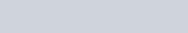Resistance is measured in ohms, which is symbolised by the Greek letter Omega (Ω). In the honour of Georg Simon ohm, it’s SI unit is ohm.

#### DEFINITION OF ONE OHM

The resistance of the conductor is said to be one ohm, if one ampere of electric current is flows when a potential difference of one volt is applied across the end of the conductor.

Dimensional formula of the electrical resistance is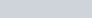.

#### FACTORS AFFECTING RESISTANCE

Every material have the property of resistance. But there are some material which didn’t oppose the motion of the electrons.

These materials offers zero resistance to the electric current. The materials which offers zero resistance to the electric current are called superconductor.  Some examples are : aluminium, niobium, magnesium diboride, cuprates such as yttrium barium copper oxide and iron pnictides.

The materials which have high conductivity have the lower resistance whereas the materials which have lower conductivity have the higher resistance.

Resistance of the conductor depends on the many factors. Some affecting factors are discussed below :

1). Resistance of the conductor is directly proportional to the length of the conductor. i.e

(1)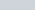It means that the length of the conductor increases then the resistance of that conductor also increases.

Why it happens? It happens because when the length increases, the interval of the collision between the atoms and molecules will be longer.

2). It is inversely proportional to the area of the cross section of the conductor. i.e

(2)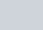It means that if cross sectional area of the conductor increases then the value of the electric resistance decreases.

Why it happens? It happens because if cross sectional area of the conductor is large then the electrons have the large space to travel inside. This large space minimise the collision between the atoms and molecules and this lead to the lower resistance. Combining equ (1) and 2, we get-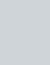Where ρ is a constant of proportionality and also called the resistivity of the conductor. The values of ρ depend on the nature (atomic structure) of the materials.

3). Resistance of the conductor depends on the nature of the material (atomic structure) of which conductor is made.

4). Resistance of the conductor also depends on the temperature of the conductor.

Let’s consider a conductor whose resistance at tempt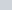is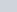and resistance at tempt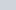is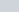. The relation between temperature and resistance is approximately given as-Where α is the temperature coefficient of the resistance andis the resistance at zero degree temperature. Andis the resistance at.

Thus, temperature coefficient of resistance is defined as the increase in resistance per unit original resistance per degree Celsius or kelvin rise in tempt.

Here you can clearly see that resistance of any conductor due to temperature depends of the following three factors.

• Initial temperature of the conductor.
• Temperature rise in the conductor.
• Temperature coefficient of the resistance.

FOR METALS : The value of α is positive , therefore resistance of the metals increases with rise of temperature.

FOR INSULATORS AND SEMICONDUCTOR : The value of α is negative, therefore resistance decreases with the rise of temperature.

FOR ALLOYS : The value of α is very small.

## RESISTIVITY OR SPECIFIC RESISTANCE

### DEFINITIONSpecific resistance or resistivity of the material of a conductor is defined as the resistance due to unit length with unit area of cross section of the material of the conductor.

It is also defined as the resistance of unit cube of a material of the given conductor.

If we take length = 1 unit and cross sectional area = 1 unit, then substituting it into the formula of resistivity, it becomes-### UNIT

we know that, resistance of any conductor depends as follows :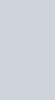Put the units of all the quantities which is in the formula of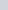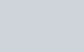Unit of resistivity is ohm-metre or Ωm and it’s dimensional formula is.

### DEFINITION OF ONE OHM-METRE RESISTIVITY

Resistivity of a conductor is said to be one ohm-metre, if one ohm of resistance is caused by a conductor whose length is one metre and it’s cross sectional area is one metre square.
Mathematically,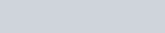### MICROSCOPIC FORM OF RESISTIVITY

Till now, we have discussed resistivity at macroscopic level. But we can convert formula of resistivity in the form of microscopic entities i.e electrons.
Mathematically,Put value of R in above formula of resistivity, we get-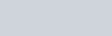Therefore, resistivity of the conductor is-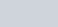### FACTORS AFFECTING RESISTIVITY

From the above formula, it is clear that resistivity of a conductor depends on the following factors:

•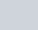, i.e., resistivity of a conductor is inversely proportional to the density of free electrons (numbers of free electrons per unit volume), as we know that free electrons inside any conductor depends on the nature of the material of the conductor, so resistivity of a conductor depends on the nature of the material.
•, i.e., resistivity of a material is inversely proportional to the relaxation time of the free electrons of the conductor. As value ofdepends on the temperature of the conductor, so resistivity of a conductor is also depends on the temperature of the conductor, if temperature increases,decreases and henceincreases.

### EFFECTS OF TEMPERATURE ON RESISTIVITY

We have seen the effect of temperature on resistivity. If temperature increases then the resistivity increases because relaxation time decreases.

Resistivity is a special case of resistance, so it is called specific resistance. It’s relationship with the temperature is same as the relationship of resistance with temperature.
Resistivity of a conductor in terms of temperature is given by-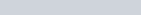Whereis the resistivity at temperature T and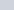is the resistivity at temperature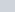andis temperature coefficient of resistivity.Thus, temperature coefficient of electrical resistivity is also defined as the fractional change in electrical resistivity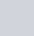per unit change in temperature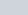FOR METALS : The value ofis positive, therefore resistivity of conductors increases with increase in temperature.

FOR SEMICONDUCTORS : Resistivity decreases with increase in temperature.

FOR ALLOYS : Resistivity of almost all alloys increases with the rise of temperature but rate of change resistivity in alloys is less than that of pure metals.

In fact, the resistance of certain alloys such as Manganin, Eureka, and Constantan show practically no change in resistance in a considerable range of temperature.

Here a live illustration of dependency of resistance on the resistivity, length and cross sectional area. Enjoy and understanding the how they are related to each other.